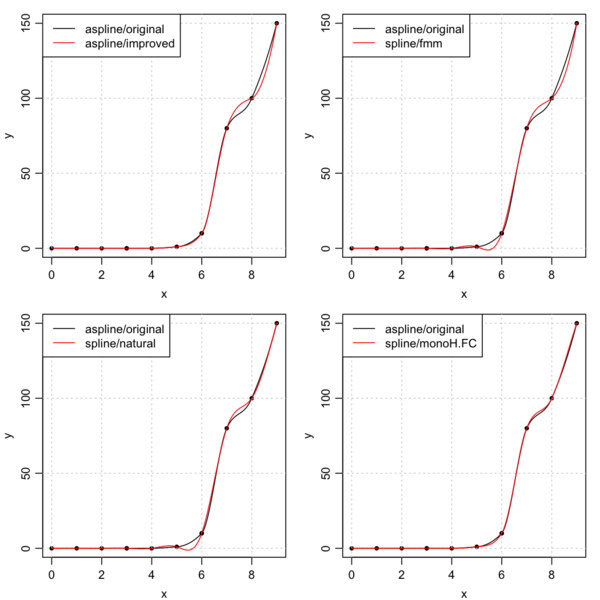# Introduction

This follows up on the previous posting, using data provided by Akima (1972). The four panels of the plot produced by the following code compare the original Akima spline formula with an improved version, and three styles of `spline()` fits.

As noted in the code, two `spline()` methods are useless for general tasks and are ignored here. The `"period"` method sets the endpoint y-values equal, which will be useless in most applications. The `"hyman"` method requires the y values to be increasing, which again is useless in most applications.

# Methods

The code first creates the data, the MD=1 case of Akima (1972), and defines `xx` as a finer-resolution version of `x` for plotting. Then it defines a function `start` for common operations on each panel, and then it displays comparisons. The idea of showing each in reference to the original akima method is to guide the eye, since plotting all in one panel makes a tangle that is difficult to grasp.

# Results# Conclusions

1. `spline()` with `method="natural"` or `"fmm"` produces undesirable wiggles (for x between 5 and 6).

2. `spline()` with `method="monoH.FC"` produces results that re very similar to `aspline()` with `method="original"`.

3. Arguably, `aspline()` with `method="original"` produces results most similar to what one might draw by hand; as just noted, `spline()` with `method="monoH.FC"` produces essentially equivalent results.

4. It would be a bad idea to base general selection on just this one test, but the code provided here is easy to modify for real data (alter lines 5 and 6), so this posting may be useful to readers.

Hiroshi Akima, 1972. Algorithm 433 Interpolation and smooth curve fitting based on local procedures (E2). Communications of the Association for Computing Machinery, 15(10): 914-918.